chapter 1 quiz 2 practice
period *
Angle A and B are complementary. <A = 8x - 18 and <B = 4x + 12. Find the measure of angle A. *
1 point
Find measure of angle x. *
1 point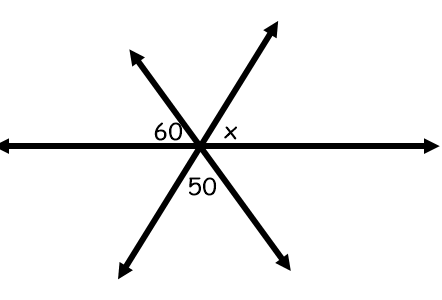solve for x *
1 point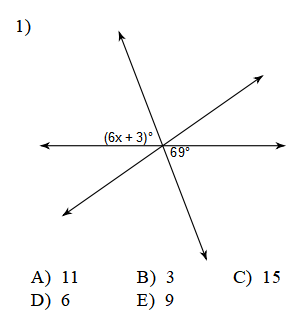Find angle x. *
1 point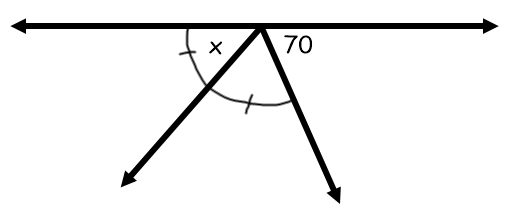solve for x *
1 point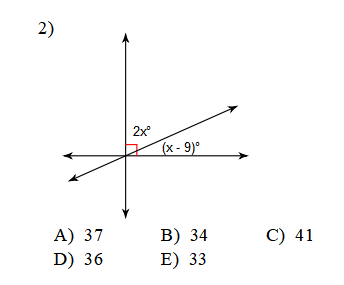Find angle x. Just give me the number. if you think x is 90, just put 90 *
1 point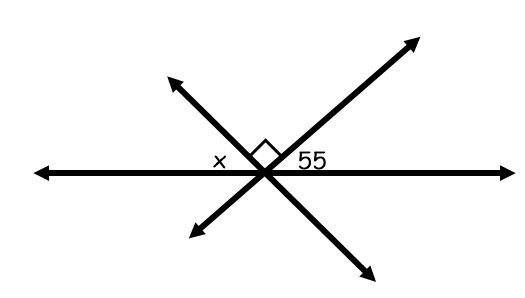solve for x *
1 point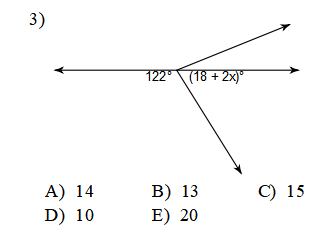last name *
Find measure of angle x. Just give me the number. nothing else will work. *
1 point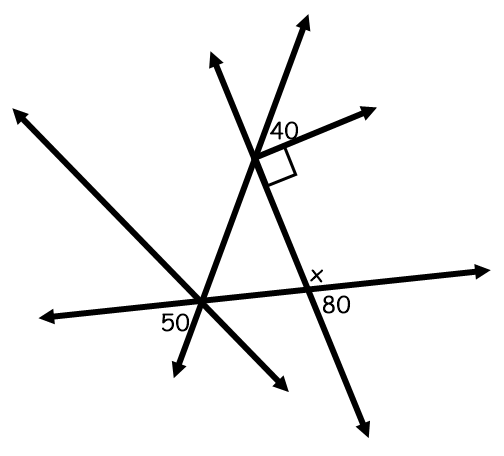Never submit passwords through Google Forms.
This content is neither created nor endorsed by Google. Report Abuse - Terms of Service - Privacy Policy